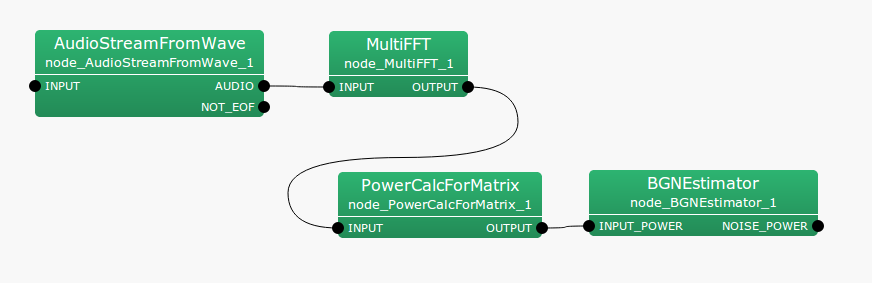6.7.23 PowerCalcForMatrix

6.7.23.1 Outline of the node

This node converts Matrix<complex<float> > type multichannel complex spectra into the real power/amplitude spectra.

6.7.23.2 Necessary files

No files are required.

6.7.23.3 Usage

When to use

This node is used to convert complex spectra input as Matrix<complex<float> > type into real power/amplitude spectra. When inputs are of Map<int, ObjectRef> type, use the PowerCalcForMap node.

Typical connection

Figure 6.128 shows an example of the usage of a PowerCalcForMatrix node. A Matrix<complex<float> > type complex spectrum obtained from the MultiFFT node is converted into a Matrix<float> type power spectrum and input to the BGNEstimator node.Figure 6.128: Example of a connection of PowerCalcForMatrix

6.7.23.4 Input-output and properties of the node

Table 6.123: Parameters of PowerCalcForMatrix
 Parameter name Type Default value Unit Description POWER_TYPE POW Selection of power/amplitude spectra

Input

INPUT

: Matrix<complex<float> > type. A matrix with each element as a complex number.

Output

OUTPUT

: Matrix<float> type. A real matrix consisting of power/absolute values of each element of the input.

Parameter

POWER_TYPE

: string type. Selection of power (POW) or amplitude (MAG) spectra for the output.

6.7.23.5 Details of the node

The real matrix $N_{i,j}$ of the output of the input complex matrix $M_{i,j}$ ($i, j$ indicating the rows and columns of the index, respectively) can be obtained as follows.

 $\displaystyle N_{i,j}$ $\displaystyle =$ $\displaystyle M_{i,j}M_{i,j}^*~ ~ (\textrm{if POWER\_ TYPE=POW}),$ $\displaystyle N_{i,j}$ $\displaystyle =$ $\displaystyle abs(M_{i,j})~ ~ (\textrm{if POWER\_ TYPE=MAG}),$

Here, $M_{i,j}^{*}$ indicates the complex conjugate of $M_{i,j}$.